# 电磁场与电磁波pdf/doc/txt格式电子书下载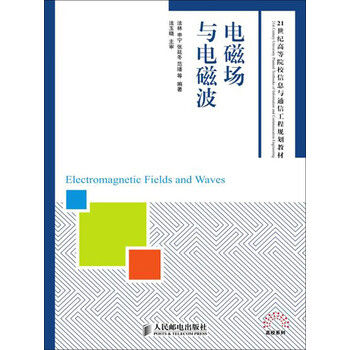ISBN：9787115293220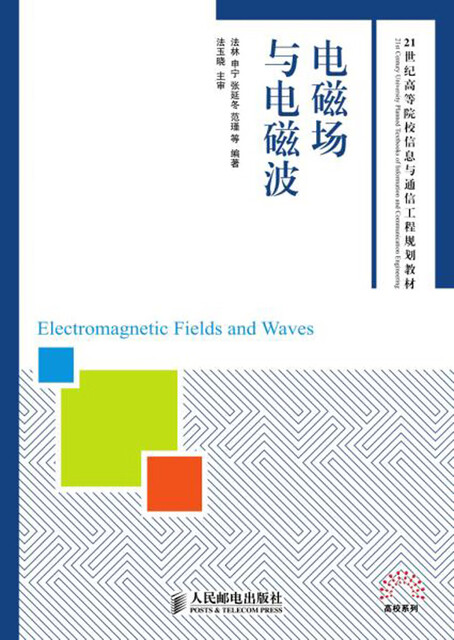# 前言

“电磁场与电磁波”是高等院校电子信息类专业的一门重要基础课，其理论涉及通信、雷达、遥感、导航、电子对抗、地球物理等各个领域。本门课程是学生在学完“高等数学”、“大学物理”和“数学物理方程”等课程之后开设的后续课程，通过该门课程的学习，可以使学生掌握电磁场与电磁波的基本理论，学会用“场”的思想分析和解决问题，提高学生的抽象思维能力和科技创新能力。

（1）根据编者的教学经验和体会，先学习静态场，学生更容易学习时变场的内容。以亥姆霍兹定理为理论依据，给出一个整体框架，使学生明白每章所要学习的内容，通过静态场的学习，引出时变场的麦克斯韦方程组，给出静态场是时变场的特例。

（2）不同于大多数的教材，本书去掉了导行电磁波与电磁辐射的内容。这主要是基于48学时课程的考虑，并且“微波技术基础”和“天线与电波”等后续课程中都有此内容的详细阐述。

（3）在内容安排上注意重点和难点问题，或一般教材中讲述较少的内容。例如：从物理概念上清楚阐述了感生电动势和动生电动势的相对关系，从而可以加深对麦克斯韦第二方程的理解。

（4）在内容讲解上，对全书核心内容的麦克斯韦方程组的各个方程都做了详细的推证和讲解。

（5）本书注重教学内容和实际应用相结合，例如文中给出了磁偶极子在石油测井中的应用实例。

2012年7月9日

# 第1章 矢量分析

## 1.1 标量场和矢量场

### 1.1.2 矢量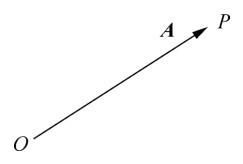Α=AeA　　　　　　　　　　　　(1.1.1)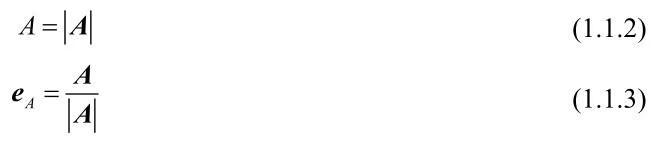## 1.2 矢量运算

### 1.2.1 矢量加法

C=Α+B　　　　　　　　　　　　　　(1.2.1)

C=Α+B=B+Α　　　　　　　　　　　　(1.2.2)

Α+B+C+D=(Α+B)+(C+D)=Α+(B+C)+D　　　　　　(1.2.3)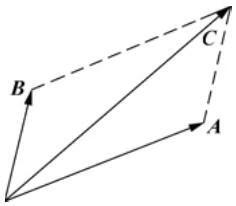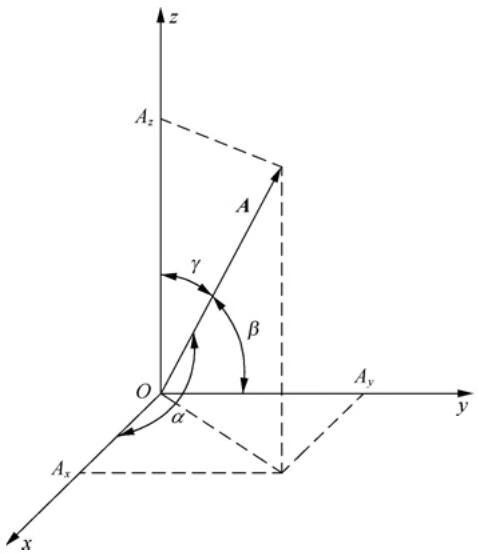Α=Αxyz　　　　　　　　　　　(1.2.4)

Α=exAx+eyAy+ezAz　　　　　　　　　　(1.2.5)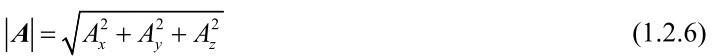Α的单位矢量eA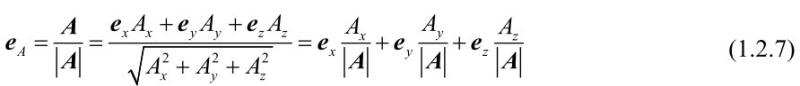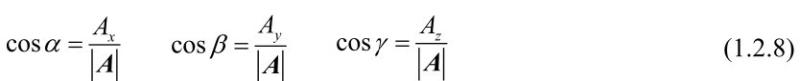Α=exAx+eyAy+ezAz　　　　　　　　　　(1.2.9)

B=exBx+eyBy+ezBz　　　　　　　　　　(1.2.10)

C=exCx+eyCy+ezCz　　　　　　　　　　(1.2.11)

Α+B+C=ex(Ax+Bx+Cx)+ey(Ay+By+Cy)+ez(Az+Bz+Cz)　　　(1.2.12)

### 1.2.2 矢量减法

Α−B=Α+(−B)　　　　　　　　　　　　(1.2.13)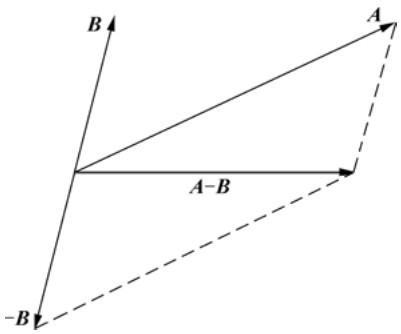### 1.2.4 两矢量的标量积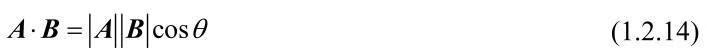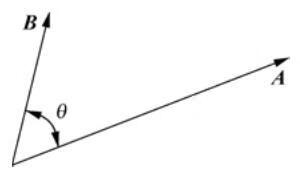Α· B=0　　　　　　　　(1.2.15)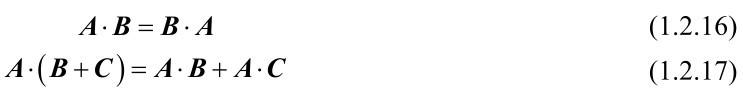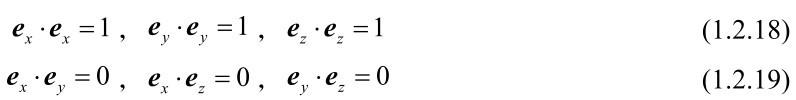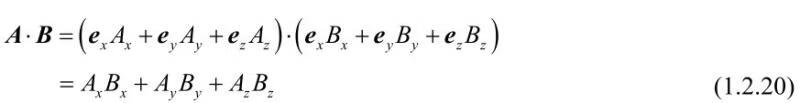### 1.2.5 两矢量的矢量积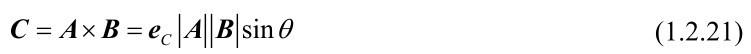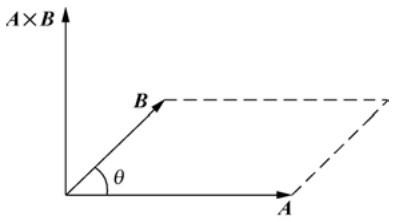Α×B=−B×Α　　　　　　　(1.2.22)

Α×(B+C)=Α×B+Α×C　　　　(1.2.23)

(Α×B)×C≠Α×(B×C)　　　　　　　　(1.2.24)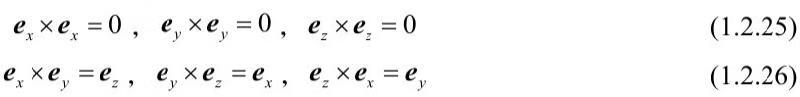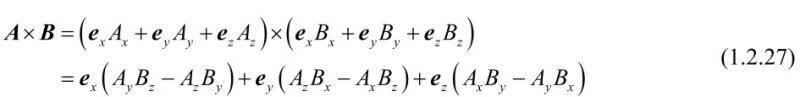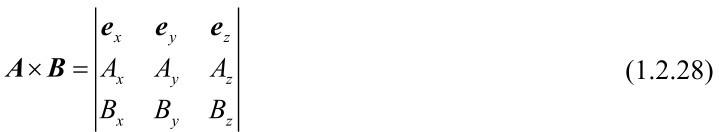### 1.2.6 矢量三重积

3个矢量Α、B和C相乘可以分为两种情况：Α·(B×C)结果是一个标量，称为标量三重积；Α×(B×C)结果是一个矢量，称为矢量三重积。在矢量运算中，规定先进行叉积运算，后进行点积运算。

Α·(B×C)=B·(C×Α)=C·(Α×B)　　　　　　　(1.2.29)

Α×(B×C)=(Α·C)B−(Α·B)C　　　　　　　(1.2.30)

## 1.3 3种正交坐标系

### 1.3.1 直角坐标系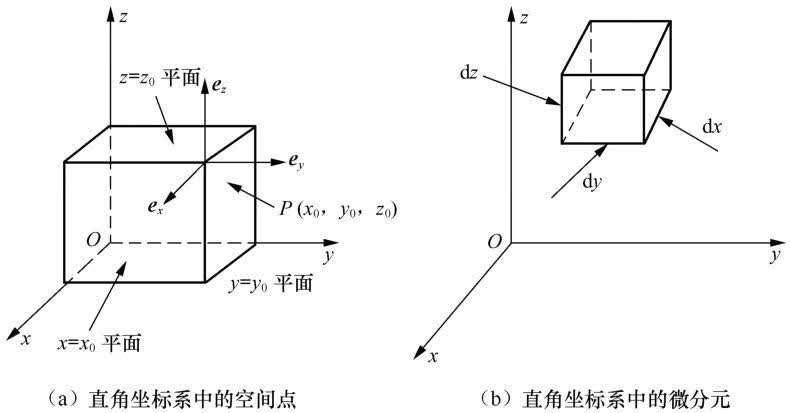（1）线元

dl=exdx+eydy+ezdz　　　　　　　　　　(1.3.1)

（2）面元

dSx=exdydz　　　　　　　　　　　　(1.3.2)

dSy=eydxdz　　　　　　　　　　　　(1.3.3)

dSz=ezdxdy　　　　　　　　　　　　(1.3.4)

（3）体积元

dV=dxdydz　　　　　　　　　　　　　(1.3.5)

### 1.3.2 圆柱坐标系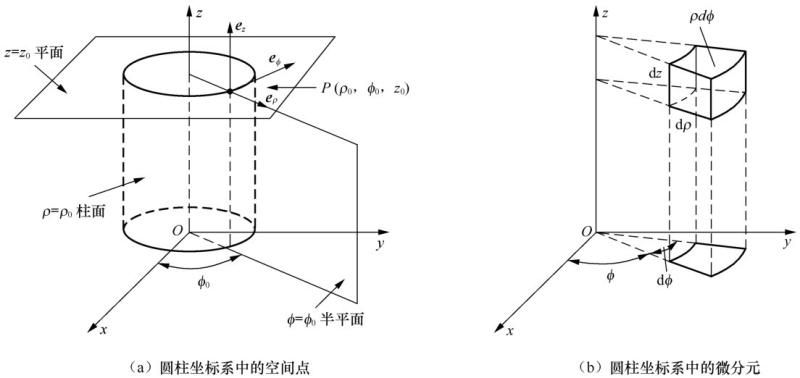（1）线元

dl=eρd ρ+eφρd φ+ezd z　　　　　　　　　　(1.3.6)

（2）面元

dSρ=eρρd φd z　　　　　　　　　　　(1.3.7)

dSφ=eφdρdz　　　　　　　　　　　　(1.3.8)

dSz=ezρdρdφ　　　　　　　　　　　(1.3.9)

（3）体积元

dV=ρdρdφdz　　　　　　　　　　　　(1.3.10)

### 1.3.3 球坐标系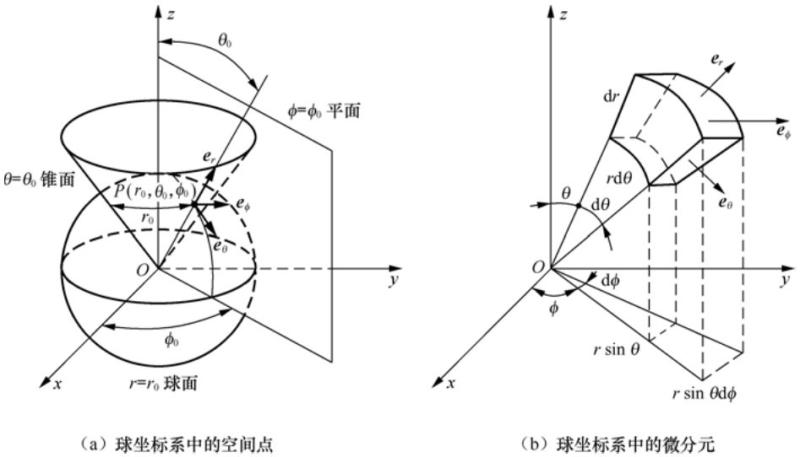（1）线元

dl=erd r+eθr dθ+eφr sinθdφ　　　　　　　　　(1.3.11)

（2）面元

dSr=err2sinθdθdφ　　　　　　　　　　(1.3.12)

dSθ=eθr sinθd r dφ　　　　　　　　　　(1.3.13)

dSφ=eφr d r dθ　　　　　　　　　　　(1.3.14)

（3）体积元

dV=r2sinθdrdθdφ　　　　　　　　　　　(1.3.15)

## 1.4 矢量在不同坐标系中的变换

### 1.4.1 圆柱坐标系与直角坐标系间的变换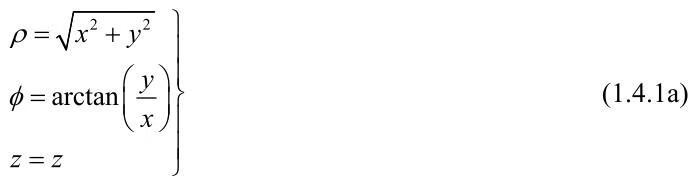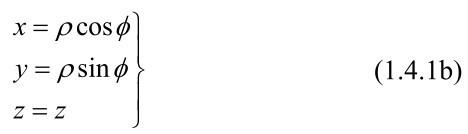Α=exAx+eyAy+ezAz　　　　　(1.4.2)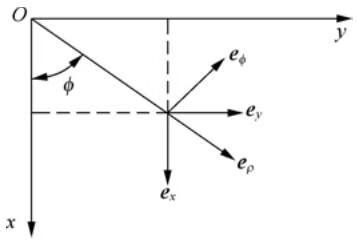Α=eρAρ+eφAφ+ezAz　　　　　　　　　　(1.4.3)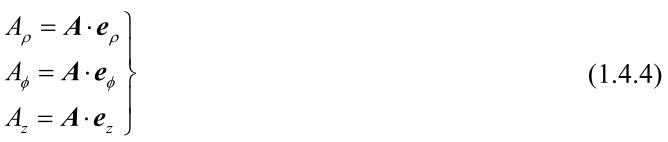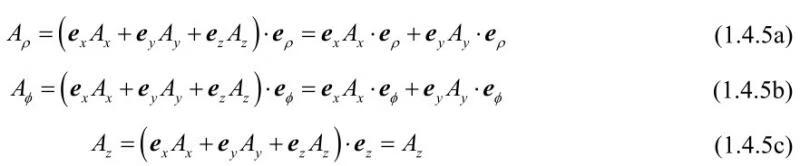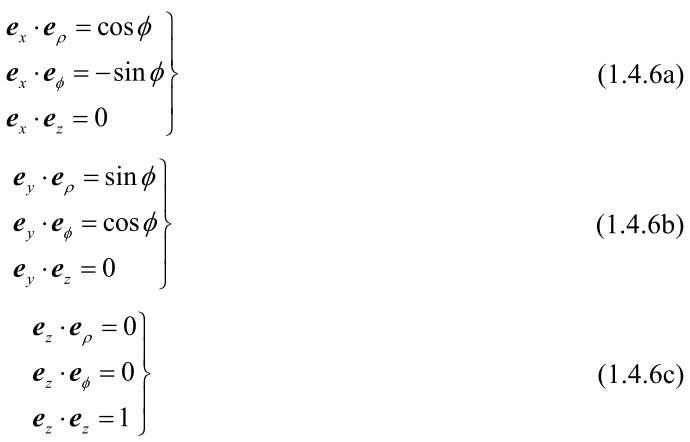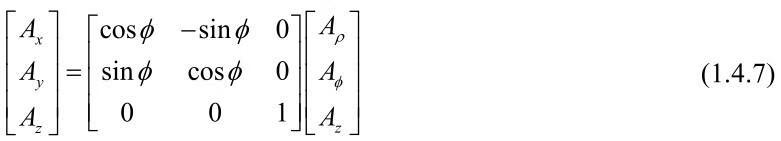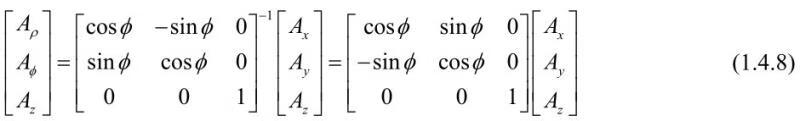【例1.4.1】 试将圆柱坐标系中的矢量Α=−eφρ+ezz变换为直角坐标系中的表达式。

Aρ=0，Aφ=−ρ，Az=z

Α=exAx+eyAy+ezAz

Ax=eφAφ· ex=ρsin φ

Ay=eφAφ· ey=−ρcos φ

Az=Az=z

Ax=y

Ay=−x

Az=z

Α=exy−eyx+ezz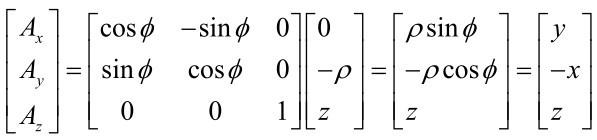Α=exy−eyx+ezz

### 1.4.2 球坐标系与直角坐标系间的变换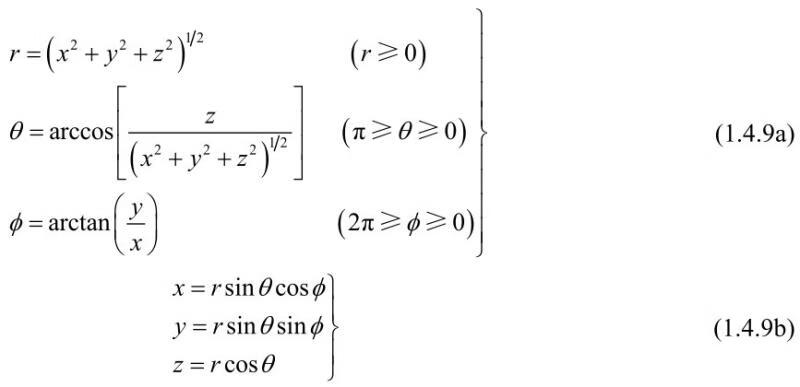Α=exAx+eyAy+ezAz

Α=erAr+eθAθ+eφAφ　　　　　　　　　　(1.4.10)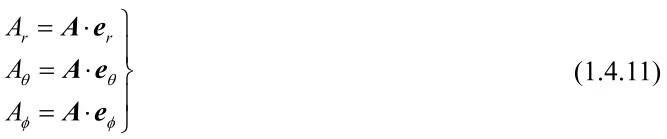Ar=Α·er=exAx·er+eyAy·er+ezAz·er　　　　　　　(1.4.12a)

Aθ=Α·eθ=exAx·eθ+eyAy·eθ+ezAz·eθ　　　　　　　(1.4.12b)

Aφ=Α·eφ=exAx·eφ+eyAy·eφ+ezAz·eφ　　　　　　　(1.4.12c)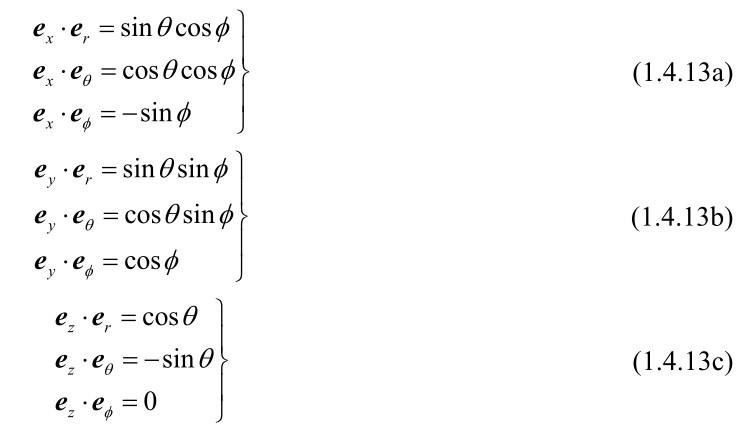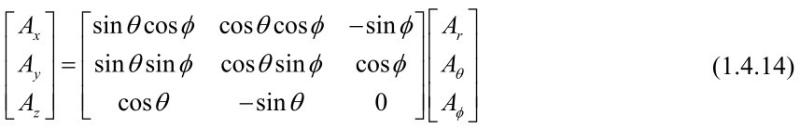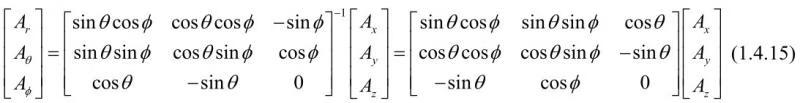【例1.4.2】 已知矢量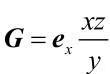，试将其变换为球坐标系的表达式。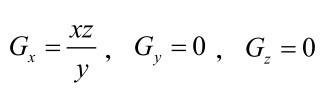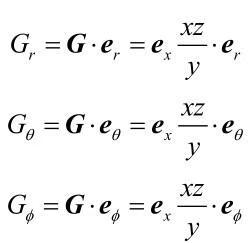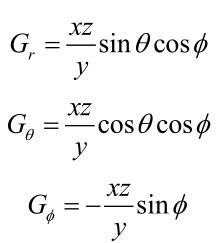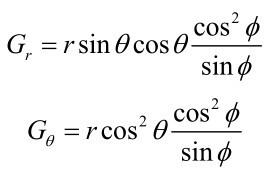Gφ=−r cosθcos φ

G=r cosθcosφ(ersinθcotφ+eθcosθcotφ−eφ)

## 1.5 标量场的梯度

### 1.5.1 方向导数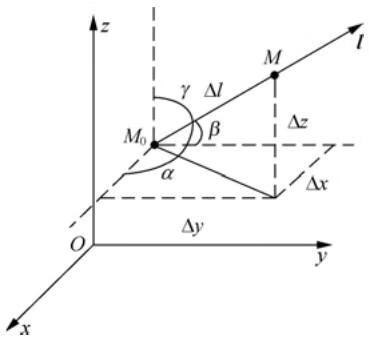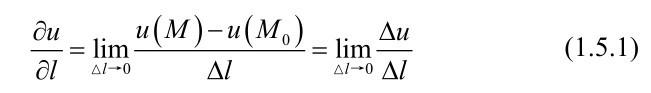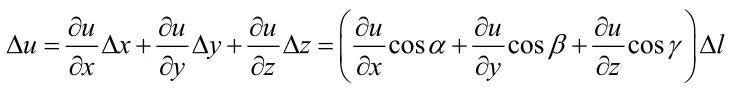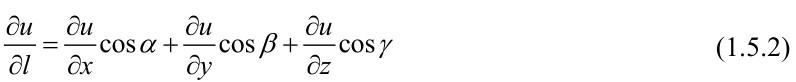### 1.5.2 梯度

el=excosα+eycosβ+ezcosγ　　　　　　　　　(1.5.3)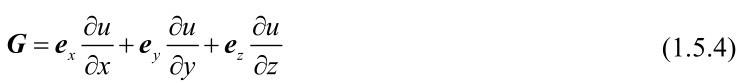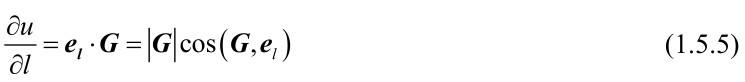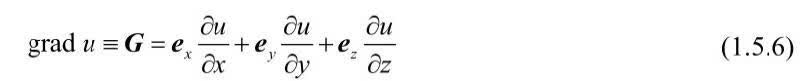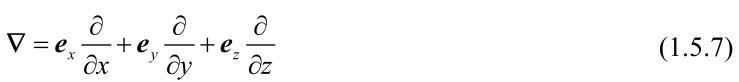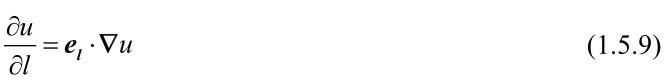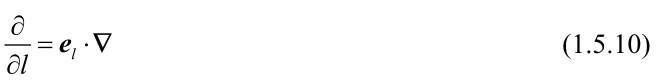u(r,t)=const　　　　　　　　　　(1.5.11)

【例1.5.1】 对标量函数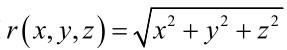，求∇r和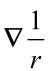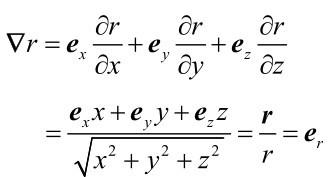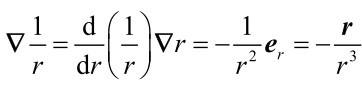【例1.5.2】 若R=r−r′、r=exx+eyy+ezz、r′=exx′+eyy′+ezz′，求∇R和∇′R。其中R=|r−r′|，∇′和∇分别对带撇和不带撇的坐标进行运算。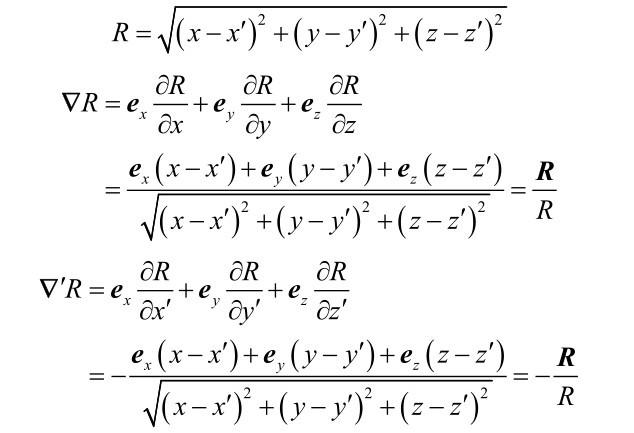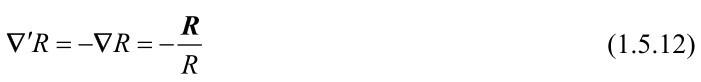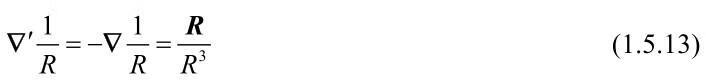【例1.5.3】 求∇e−jk·r，其中k为与坐标无关的常矢量。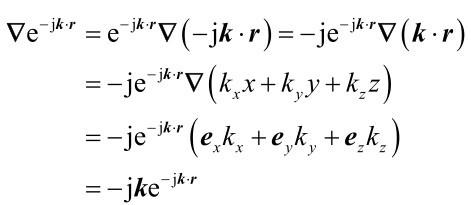## 1.6 矢量场的散度

### 1.6.1 矢量场的矢线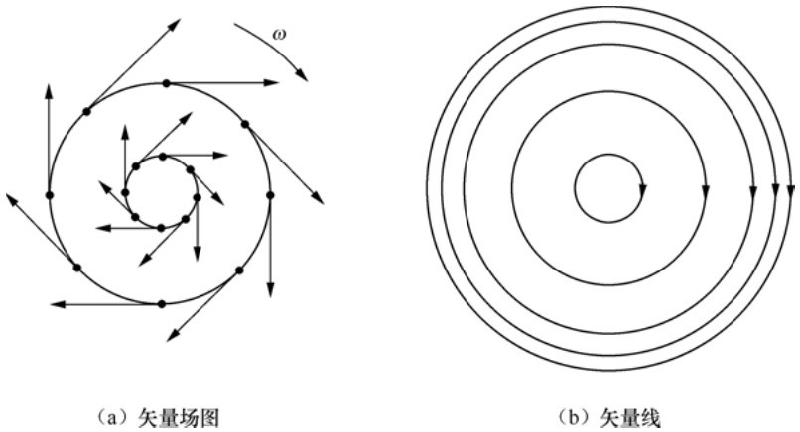### 1.6.2 通量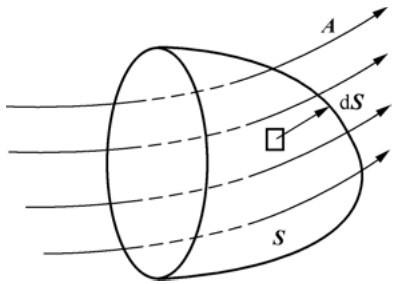dΦ=Α · dS　　　　　　　(1.6.1)

Α穿过整个曲面S的通量为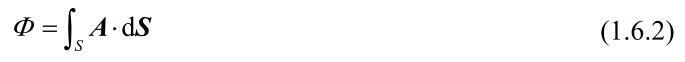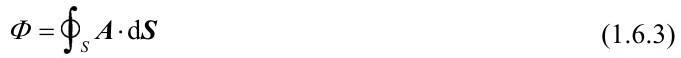### 1.6.3 散度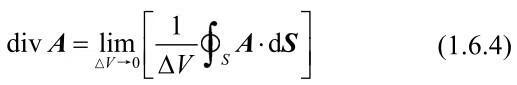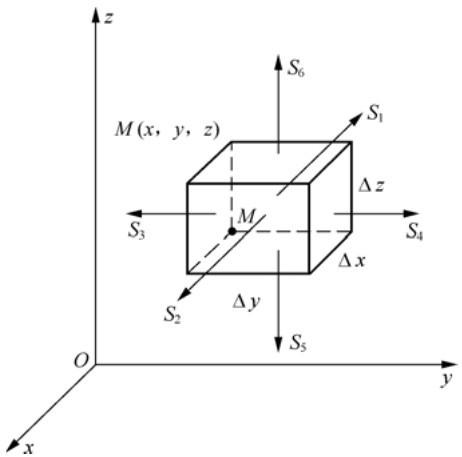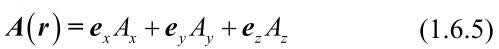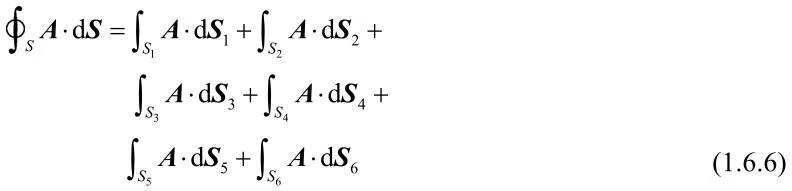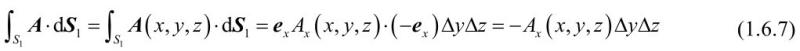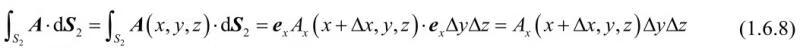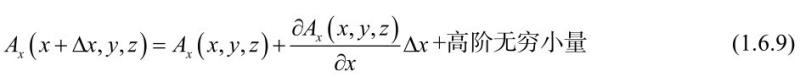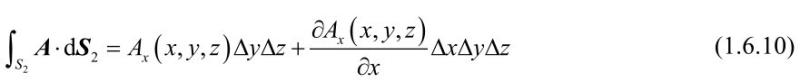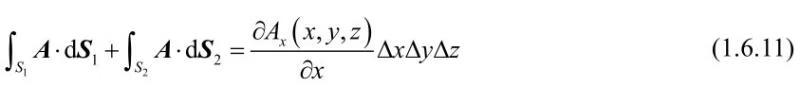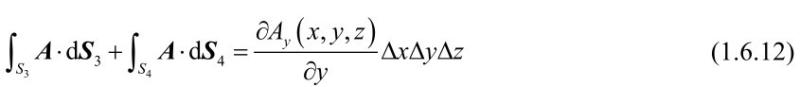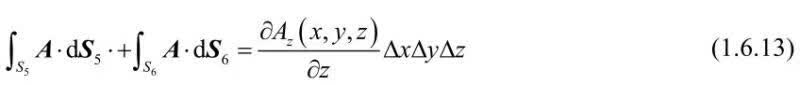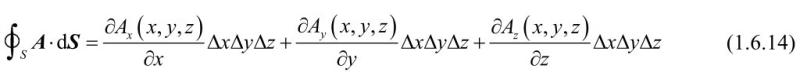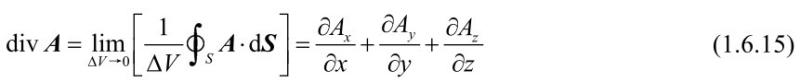### 1.6.4 散度定理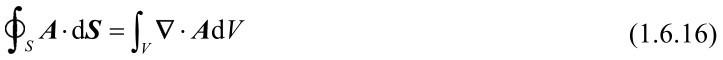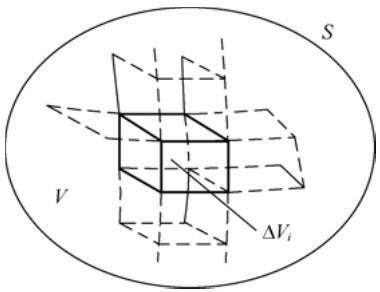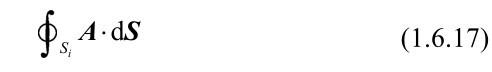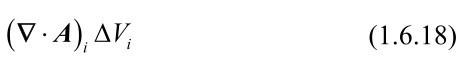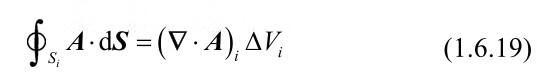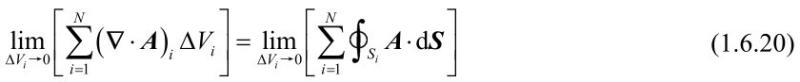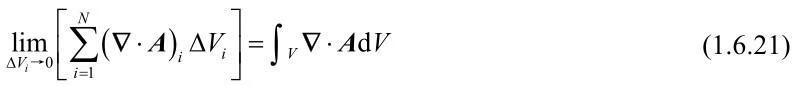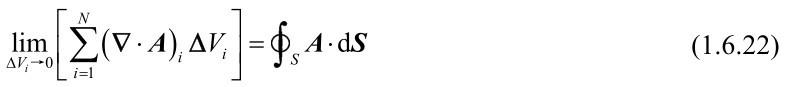【例1.6.1】 试求∇·r和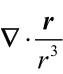，设r≠0。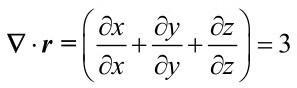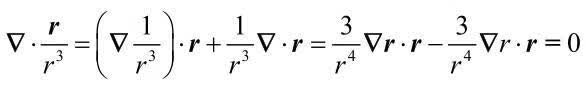【例 1.6.2】 设S为以(0,0,a)为球心，半径为R(＜a)的球面。求积分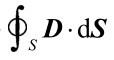，其中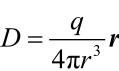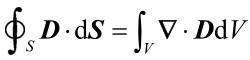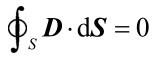## 1.7 矢量场的旋度

### 1.7.1 矢量场的环量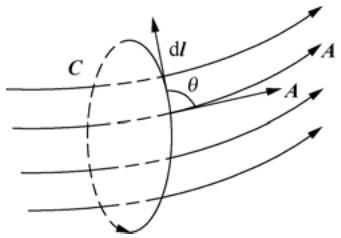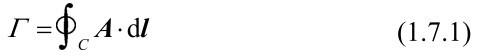....